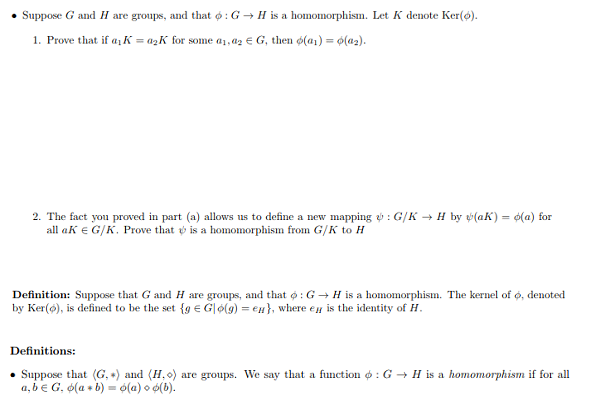# . Suppose G and H are groups, and that ф : G-> H is a homomorphism. Let K denote Ker(O).Prove that if αι A's a2K for solne α 1.126 G, then φ(al)-0(a2).1.2. The fact you proved in part (a) allows us to define a new mapping e: G/K-+ H by(aK)-d(a) forall a KG/K. Prove that ψ is a homomorphism fron G/K to HDefinition: Suppose that G and H are groups and that φ : G → H is a homomorphism. The kernel of φ, denotedby Ker(о), is defined to be the set {g Glo(g)-ell), where e" is the identity of HDefinitions:. Suppose that (G,*) and (1,0) are groups. We say that a function φ : G → H is a hornomorphism if for all

Question
18 views

Abstract Algebra:

Its a part 1 and 2 problem.

Definitions are provided.

I need some help on this problem.help_outlineImage Transcriptionclose. Suppose G and H are groups, and that ф : G-> H is a homomorphism. Let K denote Ker(O). Prove that if αι A's a2K for solne α 1.126 G, then φ(al)-0(a2). 1. 2. The fact you proved in part (a) allows us to define a new mapping e: G/K-+ H by (aK)-d(a) for all a K G/K. Prove that ψ is a homomorphism fron G/K to H Definition: Suppose that G and H are groups and that φ : G → H is a homomorphism. The kernel of φ, denoted by Ker(о), is defined to be the set {g Glo(g)-ell), where e" is the identity of H Definitions: . Suppose that (G,*) and (1,0) are groups. We say that a function φ : G → H is a hornomorphism if for all fullscreen
check_circle

Step 1

To understand homomorphism from quotient groups

Step 2
Step 3

Thus , in this example , we have shown the claim of Part...

### Want to see the full answer?

See Solution

#### Want to see this answer and more?

Solutions are written by subject experts who are available 24/7. Questions are typically answered within 1 hour.*

See Solution
*Response times may vary by subject and question.
Tagged in

### Math